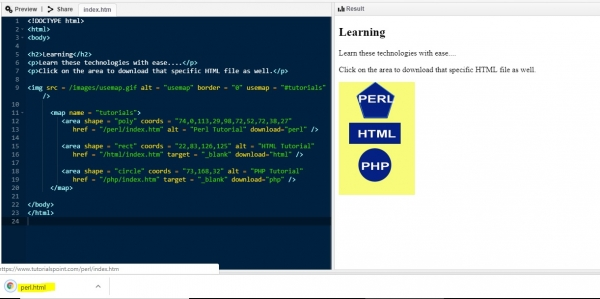HTMLWeb DevelopmentFront End Technology

Following is the syntax −

<area download="file">

Let us now see an example to implement the download attribute of the <area> element −

## Example

Live Demo

<!DOCTYPE html>
<html>
<body>
<h2>Learning</h2>
<p>Learn these technologies with ease....</p>
<img src = /images/usemap.gif alt = "usemap" border = "0" usemap = "#tutorials"/>
<map name = "tutorials">
<area shape = "poly" coords = "74,0,113,29,98,72,52,72,38,27"
<area shape = "rect" coords = "22,83,126,125" alt = "HTML Tutorial"
<area shape = "circle" coords = "73,168,32" alt = "PHP Tutorial"
</map>
</body>
</html>

## OutputNow, when you will click on let’s say “PERL”, the file will download as shown below −<area shape = "poly" coords = "74,0,113,29,98,72,52,72,38,27"
href = "/perl/index.htm" alt = "Perl Tutorial" download="perl" />
download="perl"